﻿ 三阶线性方程满足能量守恒的差分格式

# 三阶线性方程满足能量守恒的差分格式The Difference Scheme of Energy Conservation for the Third Order Linear Equation

Abstract: Based on the third-order linear conservation equation’s many conservation laws, this paper de-signed a conservation numerical scheme which can satisfy the first two conservation laws of the equation. The numerical examples show the scheme’s effectiveness and the good stability and structure preservation in long-term numerical simulation.

1. 引言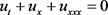(1)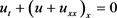(2)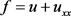(3)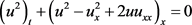(4)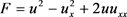(5)

2. 格式的描述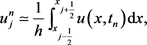(6)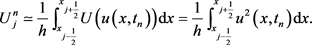(7)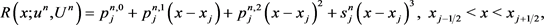(8)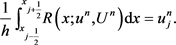(9)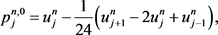(10)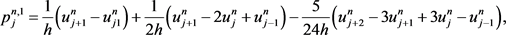(11)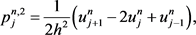(12)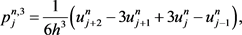(13)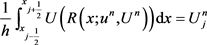(14)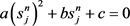(15)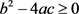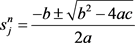(16)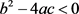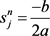(17)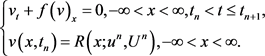(18)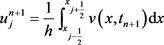(19)(20)(21)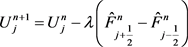(22)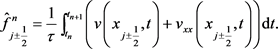(23)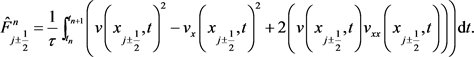(24)(25)(26)

3. 数值算例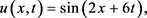(27)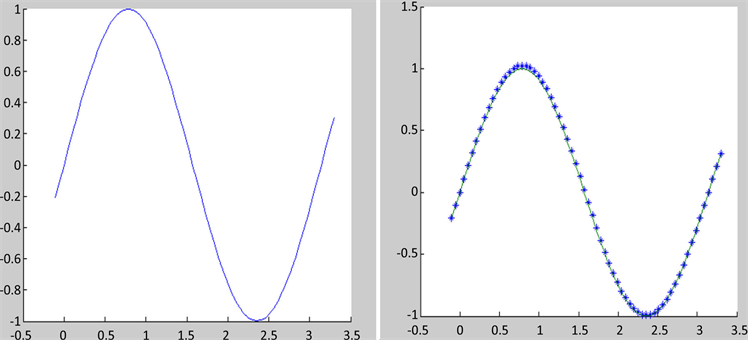(a) (b)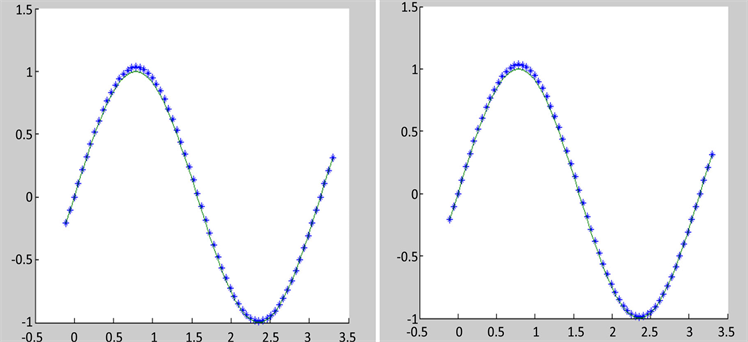(c) (d)

Figure 1. Numerical results at different times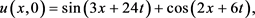(28)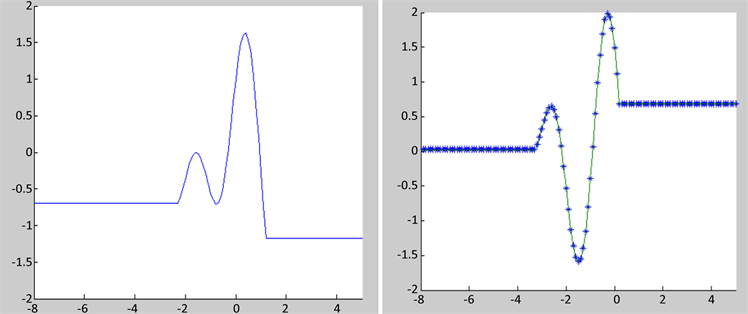(a) (b)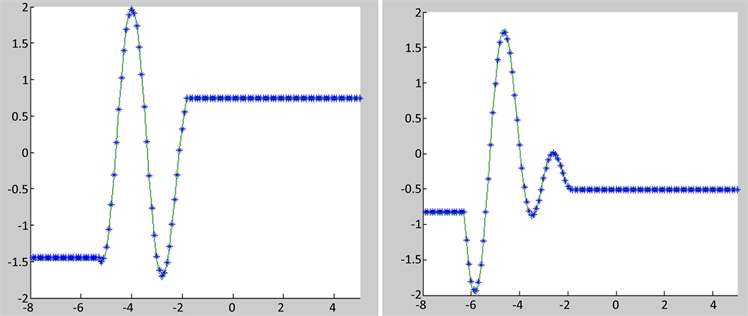(c) (d)

Figure 2. Numerical results at different times

4. 结论

 LeVeque, R. J. (2002) Finite Volume Methods for Hyperbolic Problems. Cambridge University Press, Cambridge.
https://doi.org/10.1017/CBO9780511791253

 Harten, A., Osher, S., Engquist, B. and Chakravarthy, S.R. (1986) Some Results on Uniformly High-Order Accurate Essentially Nonoscillatory Schemes. Applied Numerical Mathematics, 2, 347-377.
https://doi.org/10.1016/0168-9274(86)90039-5

 Cui, Y. and Mao, D. (2007) Numerical Method Satisfying the First Two Conservation Laws for the Korteweg-De Vries Equation. Journal of Computational Physics, 227, 376-399.
https://doi.org/10.1016/j.jcp.2007.07.031

 李红霞, 茅德康. 单个守恒型方程熵耗散格式中熵耗散函数的构造[J]. 计算物理, 2004, 21(3): 319-331.

 李红霞. 一维守恒型方程(组)的熵耗散格式[D]: [博士学位论文]. 上海: 上海大学, 2005.

 王志刚. 线性传输方程的满足多个守恒律的差分格式[D]: [硕士学位论文]. 上海: 上海大学, 2006.

 王志刚, 茅德康. 线性传输方程满足3个守恒律的差分格式[J]. 上海大学学报(自然科学版), 2006, 12(6): 588-592+598.

Top